# How to Check if the Number is Prime Number in Excel?

• Last Updated : 11 Aug, 2021

A prime number is a number that is greater than 1 and has no positive divisor other than 1 and the number itself.

### Approach:

To check whether the number is prime or not we have to divide the number with all the numbers between 2 and the square root of that number. And if it gives remainder 0 in any of the divisions it means that the number is a positive factor and ultimately results in a non-prime number. So if any of the divisions don’t result in the remainder 0 then it means that the number doesn’t have any positive factor and ultimately results in the Prime number.

Attention reader! Don’t stop learning now. If you are an Excel beginner (or an intermediate) and want to learn Excel, Geeksforgeeks brings the perfect course for you to start, Diving Into Excel

• Take the number
• If the number is 2 then it is a Prime number else follow the next steps
• Derive the square root of the number
• Take the upper bound of the square root obtained and then minus it by one
• Divide the number with all the numbers between 2 and the square root of the number
• If the remainder is 0 in any of the divisions then non-prime.
• Else it is the prime number

Formula:

`=IF(F6=2,"Prime",IF(AND(MOD(F6,ROW(OFFSET(\$C\$2,,,ROUNDUP(SQRT(F6),0)-1)))<>0),"Prime","Not Prime"))`

Let’s see each of these functions one by one so that it is clearly understood.

Step1: First of all check if the given number is 2 or not and if it is TRUE then print out that it is PRIME.

Step 2: If the number is not 2 then derive the square root of the given number.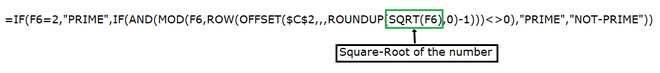Step 3: Round off the output of the square root and minus one from it ( we have to check from 2 to square root of a given number which is not included)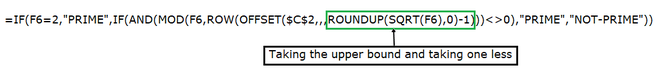Step 4: Taking the range of numbers from the 2 to the one less than the square root of the given number.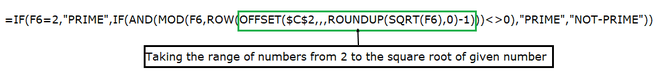Step 5: Taking one by one, the numbers from that range to evaluate further for the division.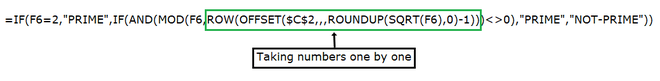Step-6: Dividing the given number from the number in the range of 2 to one less then square root of the given number and checking whether it is 0 or not. If it is 0 then the given number is NOT-PRIME and if it is not 0 then the given number is PRIME.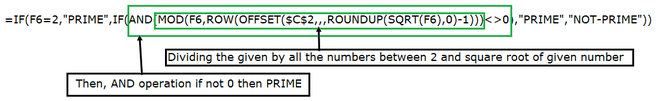Output: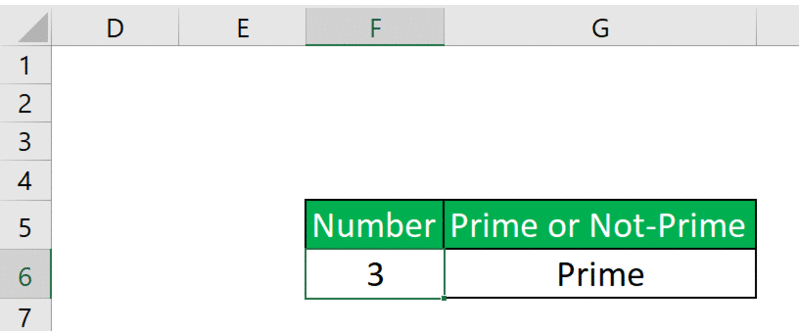My Personal Notes arrow_drop_up